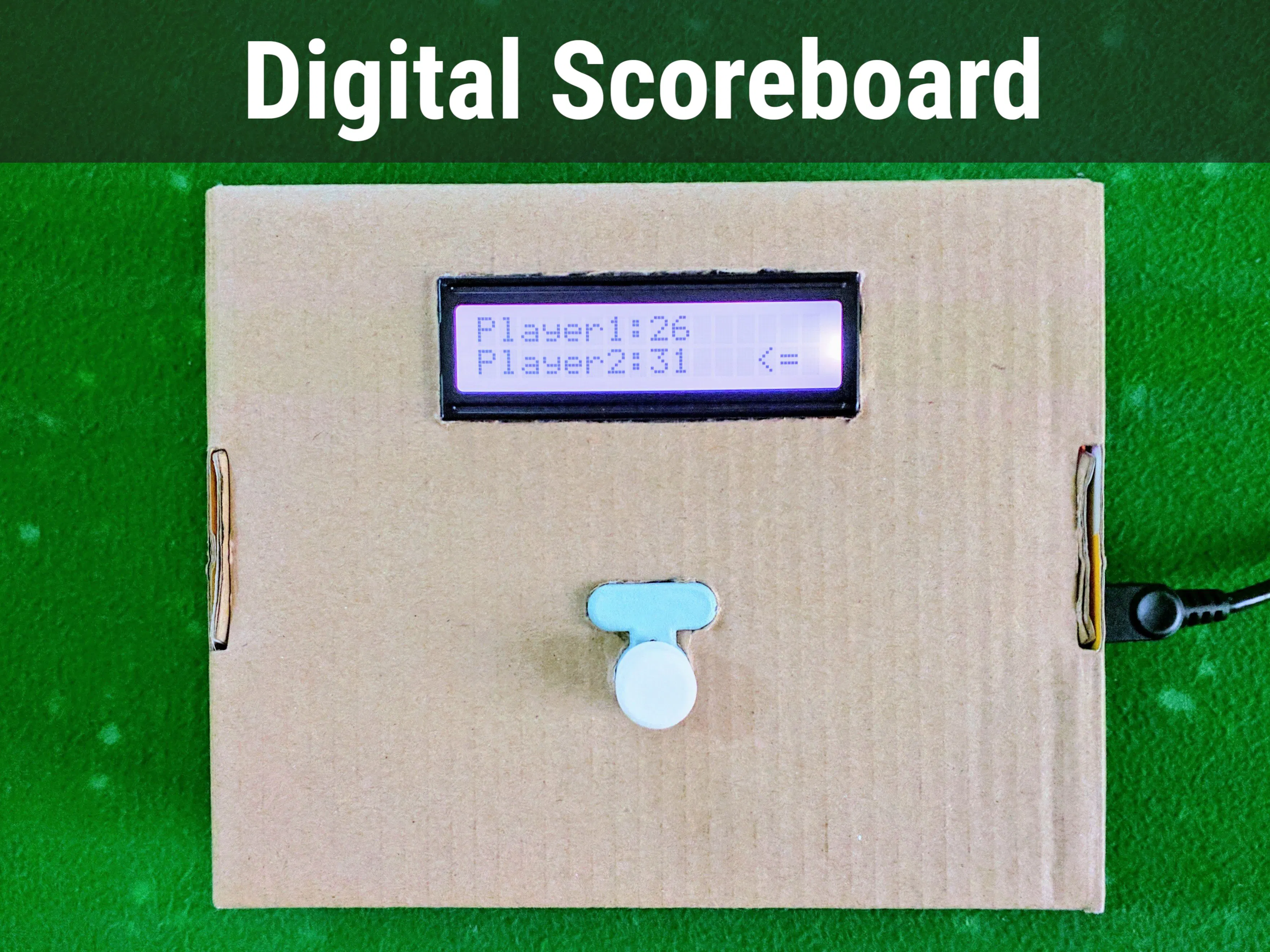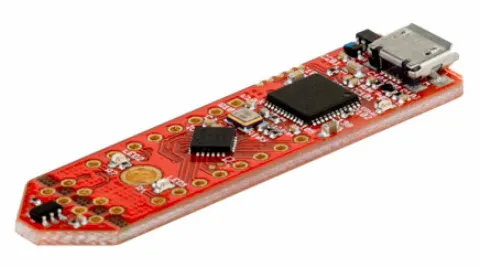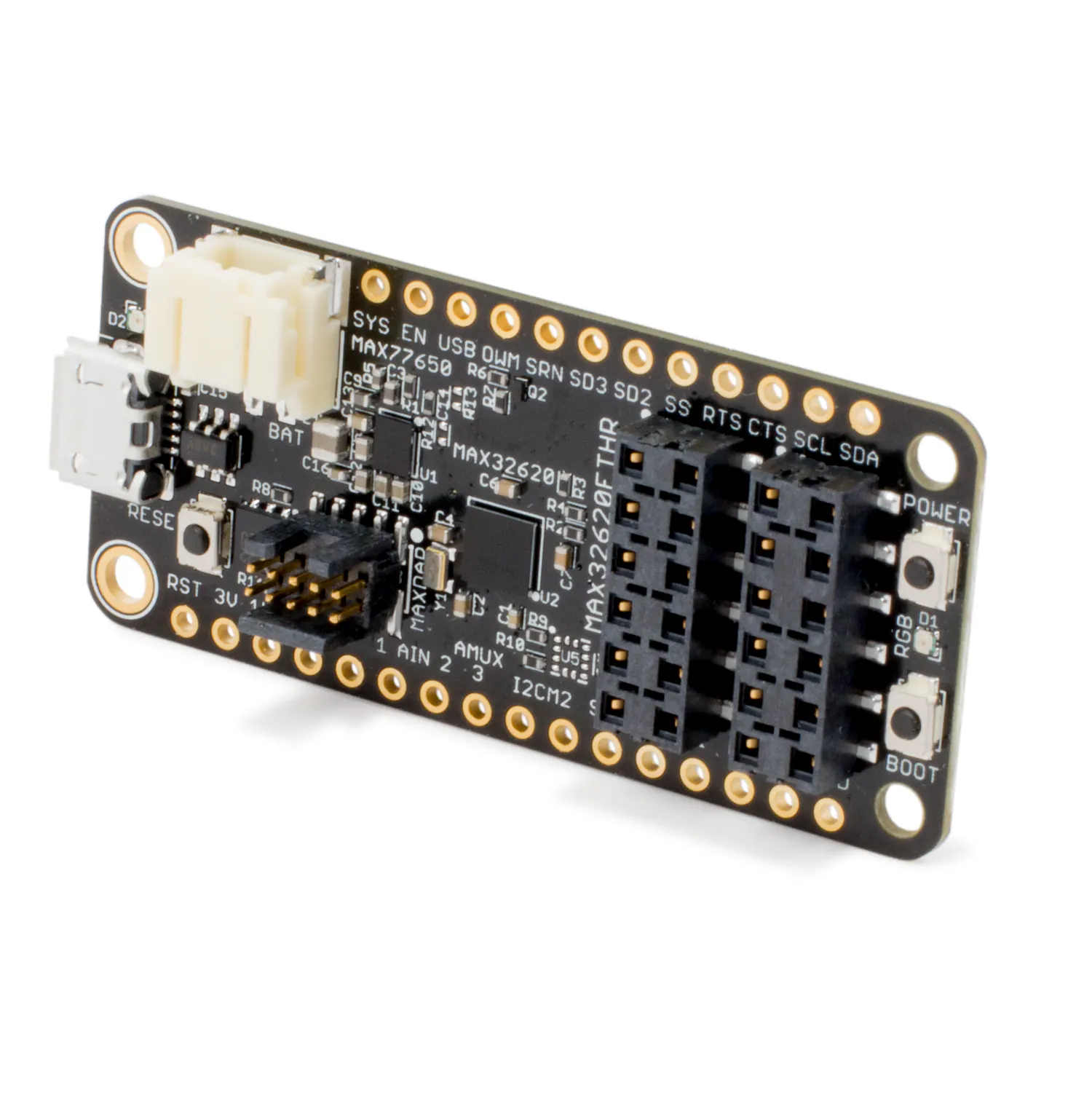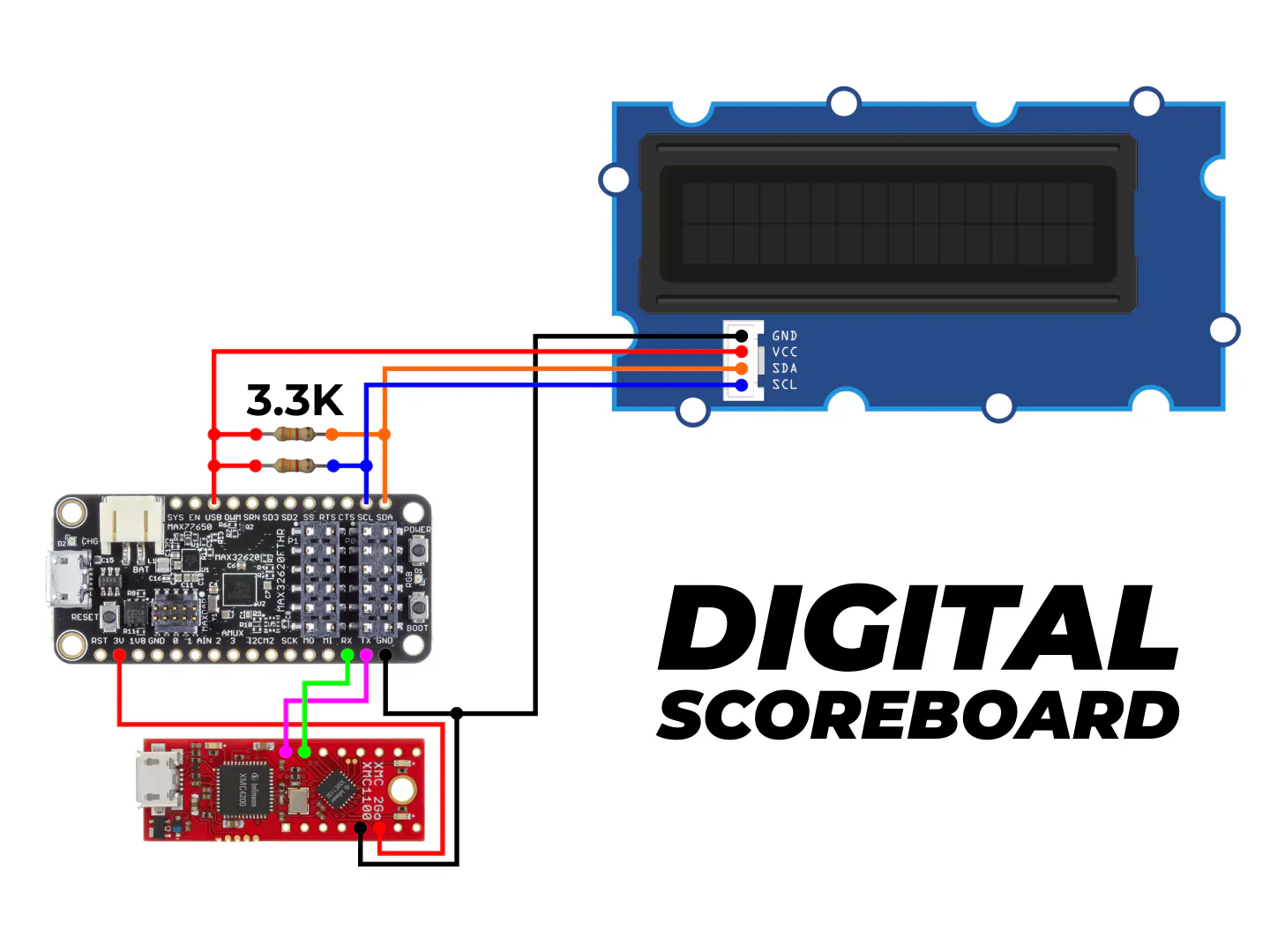# Digital Scoreboard

A digital scoreboard using the MAX32620FTHR and Infineon's 3D magnetic sensor along with the Grove LCD display.

IntermediateFull instructions provided3 hours819## Things used in this project

### Hardware componentsInfineon 3D Magnetic Sensor 2Go
×1Maxim Integrated MAX32620FTHR
×1
 Seeed Grove - LCD RGB Backlight
×1
 3.3k Resistor
×2Jumper wires (generic)
×1

### Software apps and online servicesArduino IDE

### Hand tools and fabrication machinesSoldering iron (generic)

## Schematics

### Connection Diagram

Follow this diagram to connect the MAX32620FTHR and the XMC2Go sensor kit## Code

### XMC2Go Code

Arduino
This is the code for the XMC2Go. To add more players, change the variable 'numPlayers' to whatever you want. You may also need to change the size of the arrays 'tempScore' and 'score'.
```#include <Tle493d_w2b6.h>
const float pi = (22.0 / 7.0);
float previousAngle = 0.0;
bool switchPressed;
int numPlayers = 2;   //Change this to add more players
int currentPlayer;

//If you add more players, you will need to change the size of both these arrays
float tempScore;
int score;

Tle493d_w2b6 Tle493dMagnetic3DSensor = Tle493d_w2b6();
void setup() {
Serial.begin(9600);
Tle493dMagnetic3DSensor.begin();
Tle493dMagnetic3DSensor.begin();
for (int i = 0; i < numPlayers; i++) {
tempScore[i] = 0;
score[i] = 0;
}
}

void loop() {
Tle493dMagnetic3DSensor.updateData();
delay(100);
isSwitchPressed();

float angle = pi + Tle493dMagnetic3DSensor.getAzimuth();

if (!switchPressed) {
for (int i = 0; i <= numPlayers; i++) {
if (angle > ((2 * pi) / numPlayers)*i && angle < ((2 * pi) / numPlayers) * (i + 1)) {
currentPlayer = i + 1;
}
}
} else if (switchPressed) {
//Serial.println("Switch pressed");
if (angle - previousAngle < 0) {
increaseScore();
}
else if (angle - previousAngle > 0) {
decreaseScore();
}
}

Serial.print("99999");
Serial.print(",");
Serial.print(currentPlayer);
Serial.print(",");
for (int i = 0; i < numPlayers; i++) {
Serial.print(score[i]);
if (i + 1 != numPlayers) {
Serial.print(",");
}
}
Serial.println();

previousAngle = angle;
}

void isSwitchPressed() {
if (Tle493dMagnetic3DSensor.getNorm() < 55) {
switchPressed = false;
}
else if (Tle493dMagnetic3DSensor.getNorm() > 85) {
switchPressed = true;
}
}

void increaseScore() {
for (int i = 0; i < numPlayers; i++) {
if (i + 1  == currentPlayer) {
tempScore[i] = tempScore[i] + 0.17;
score[i] = tempScore[i];
}
}
}

void decreaseScore() {
for (int i = 0; i < numPlayers; i++) {
if (i + 1  == currentPlayer) {
tempScore[i] = tempScore[i] - 0.17;
if (tempScore[i] < 0) {
tempScore[i] = 0;
}
score[i] = tempScore[i];
}
}
}
```

### MAX32620FTHR Code for 2 players

Arduino
This is the code for the MAX32620FTHR for 2 players. To modify it for more players, first make the change in the XMC2Go code, and here make more variables to hold and parse the score. Then display it as needed.
```#include <Wire.h>
#include "rgb_lcd.h"

int input;
int currentPlayer;
int score1 = 0;
int score2 = 0;

rgb_lcd lcd;

void setup()
{
// set up the LCD's number of columns and rows:
lcd.begin(16, 2);

const int colorR = 255;
const int colorG = 255;
const int colorB = 255;

Serial.begin(9600);
Serial2.begin(9600);

lcd.setRGB(colorR, colorG, colorB);
lcd.setCursor(5, 0);
lcd.print("Digital");
lcd.setCursor(3, 1);
lcd.print("Scoreboard");

delay(1700);
}

void loop()
{

input = Serial2.parseInt();
if (input == 99999) {
currentPlayer = Serial2.parseInt();
score1 = Serial2.parseInt();
score2 = Serial2.parseInt();
}

lcd.clear();
lcd.setCursor(0, 0);
lcd.print("Player1:");
lcd.print(score1);
lcd.setCursor(0, 1);
lcd.print("Player2:");
lcd.print(score2);

switch (currentPlayer) {
case 1:
{
lcd.setRGB(102, 178, 255);
lcd.setCursor(13, 0);
lcd.print("<=");
break;
}
case 2:
{
lcd.setRGB(255, 102, 102);
lcd.setCursor(13, 1);
lcd.print("<=");
break;
}
}
}
```

### MAX32620FTHR Code for 4 players

Arduino
Just an example for the scoreboard with 4 players. To use it, simply change 'numPlayers' to 4 in the XMC2Go code and upload, then upload this code to the MAX32620FTHR. Then connect everything according to the connection diagram and test.
```#include <Wire.h>
#include "rgb_lcd.h"

int input;
int currentPlayer;
int score1 = 0;
int score2 = 0;
int score3 = 0;
int score4 = 0;

rgb_lcd lcd;

void setup()
{
// set up the LCD's number of columns and rows:
lcd.begin(16, 2);

const int colorR = 255;
const int colorG = 255;
const int colorB = 255;

Serial.begin(9600);
Serial2.begin(9600);

lcd.setRGB(colorR, colorG, colorB);
lcd.setCursor(5, 0);
lcd.print("Digital");
lcd.setCursor(3, 1);
lcd.print("Scoreboard");

delay(1700);
}

void loop()
{

input = Serial2.parseInt();
if (input == 99999) {
currentPlayer = Serial2.parseInt();
score1 = Serial2.parseInt();
score2 = Serial2.parseInt();
score3 = Serial2.parseInt();
score4 = Serial2.parseInt();
}

Serial.print(currentPlayer);
Serial.print(",");
Serial.print(score1);
Serial.print(",");
Serial.print(score2);
Serial.print(",");
Serial.print(score3);
Serial.print(",");
Serial.println(score4);

lcd.clear();
lcd.setCursor(0, 0);
lcd.print("P1:");
lcd.print(score1);
lcd.setCursor(10, 0);
lcd.print("P2:");
lcd.print(score2);
lcd.setCursor(0, 1);
lcd.print("P3:");
lcd.print(score3);
lcd.setCursor(10, 1);
lcd.print("P4:");
lcd.print(score4);

switch (currentPlayer) {
case 1:
{
lcd.setRGB(102, 178, 255);
lcd.setCursor(6, 0);
lcd.print("<=");
break;
}
case 2:
{
lcd.setRGB(178, 102, 255);
lcd.setCursor(7, 0);
lcd.print("=>");
break;
}
case 3:
{
lcd.setRGB(255, 102, 102);
lcd.setCursor(6, 1);
lcd.print("<=");
break;
}
case 4:
{
lcd.setRGB(102, 255, 178);
lcd.setCursor(7, 1);
lcd.print("=>");
break;
}
}
}
```

### MAX32620FTHR code for connecting to Blynk using WIZ750SR

Arduino
To use this, just read the documentation, add your authentication code and upload to the MAX32620FTHR. This will make the scoreboard for 4 players, but to make use of it, you need to create widgets and customize them as you need in the Blynk app yourself.
```#include <Wire.h>
#include "rgb_lcd.h"
#include <BlynkSimpleStream.h>

#define vBUTTON_PIN V0
#define vP1_PIN V1
#define vP2_PIN V2
#define vP3_PIN V3
#define vP4_PIN V4

int input;
int currentPlayer;
int score1 = 0;
int score2 = 0;
int score3 = 0;
int score4 = 0;
int value;

rgb_lcd lcd;

// You should get Auth Token in the Blynk App.
// Go to the Project Settings (nut icon).
char auth[] = "YourAuthTokenHere";

void setup()
{
// set up the LCD's number of columns and rows:
lcd.begin(16, 2);

const int colorR = 255;
const int colorG = 255;
const int colorB = 255;

Serial.begin(9600);
Serial0.begin(115200);
Serial2.begin(9600);

Blynk.begin(Serial0, auth);

lcd.setRGB(colorR, colorG, colorB);
lcd.setCursor(5, 0);
lcd.print("Digital");
lcd.setCursor(3, 1);
lcd.print("Scoreboard");

delay(1700);
}

void loop()
{
Blynk.run();
input = Serial2.parseInt();
if (input == 99999) {
currentPlayer = Serial2.parseInt();
score1 = Serial2.parseInt();
score2 = Serial2.parseInt();
score3 = Serial2.parseInt();
score4 = Serial2.parseInt();
}

Serial.print(currentPlayer);
Serial.print(",");
Serial.print(score1);
Serial.print(",");
Serial.print(score2);
Serial.print(",");
Serial.print(score3);
Serial.print(",");
Serial.println(score4);

lcd.clear();
lcd.setCursor(0, 0);
lcd.print("P1:");
lcd.print(score1);
lcd.setCursor(10, 0);
lcd.print("P2:");
lcd.print(score2);
lcd.setCursor(0, 1);
lcd.print("P3:");
lcd.print(score3);
lcd.setCursor(10, 1);
lcd.print("P4:");
lcd.print(score4);

switch (currentPlayer) {
case 1:
{
lcd.setRGB(102, 178, 255);
lcd.setCursor(6, 0);
lcd.print("<=");
break;
}
case 2:
{
lcd.setRGB(178, 102, 255);
lcd.setCursor(7, 0);
lcd.print("=>");
break;
}
case 3:
{
lcd.setRGB(255, 102, 102);
lcd.setCursor(6, 1);
lcd.print("<=");
break;
}
case 4:
{
lcd.setRGB(102, 255, 178);
lcd.setCursor(7, 1);
lcd.print("=>");
break;
}
}
}

BLYNK_WRITE(vBUTTON_PIN) {
value = param.asInt(); // Get value as integer
}
```